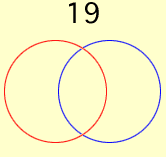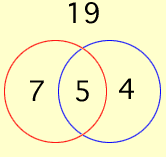Sender: Craig Bruzzone A class of 35 students took a math test and a science test. 12 students got 100% on the math test. 9 students got 100% on the science test. There were 19 students who made less than 100% on both tests. How many students made 100% on both tests? This is a sixth grade question for my daughter. Could you explain the answer. Thanks. Craig for Chelsea Bruzzone Hi Craig and Chelsea, I would do this problem with a Venn Diagram. Draw a rectangle to represent the class of 35 students and two overlapping circles inside to represent the students who got 100% in science and 100% in math. In my diagram the red circle contains the students who got 100% in math and the blue circle contains the students who got 100% in science. Since 19 students are in neither circle I wrote 19 in the rectangle but outside the circles.There are 35 students in the class so 35 - 19 = 16 students are inside the two circles. Of these 16 students 12 got 100% in math and 9 got 100% in science. But 12 + 9 = 21 which is 5 more than 16. This seeming extra 5 arises because the students who got 100% in both math and science got counted twice, once because they got 100% in math and once because they got 100% in science. Hence, in the diagram, the overlap in the circles contains 5 students.Since 12 students got 100% in math there were 12 - 5 = 7 who got 100% in math but not in science. Likewise 4 got 100% in science but not math. I hope this helps, Penny Go to Math Central